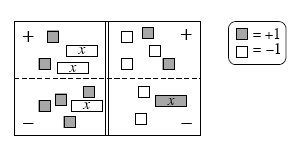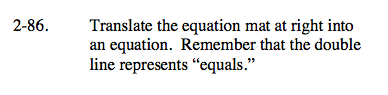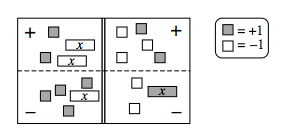### Home > CAAC > Chapter 2 > Lesson 2.1.9 > Problem2-86

2-86.

Translate the equation below into an equation. Remember that the double line represents “equals.” Homework Help ✎Use the eTool below to translate the Equation Mat into an equation.
Click the link at right for the full version of the eTool: 2-86 HW eTool.

Write the equation.

2 − 2x − (− x + 4) = 2 − 3 − (x − 2)

One strategy is to flip all of the terms in the (−) region into the (+) region, and then simplify.

2 + (−2x) − 4 + x = (−3) + 2 + 2 + (−x)
−2 + (−x) = 1 +(−x)